EnchantedLearning.com is a user-supported site.
As a bonus, site members have access to a banner-ad-free version of the site, with print-friendly pages.

 You might also like: U: Illustrated Math Dictionary - Enchanted Learning.com Y: Illustrated Math Dictionary - Enchanted Learning.com Q: Illustrated Math Dictionary - Enchanted Learning.com B: Illustrated Math Dictionary - Enchanted Learning.com I: Illustrated Math Dictionary - Enchanted Learning.com Today's featured page: Color Words: Write a Question for Each Answer

 +, - EnchantedLearning.comMath Dictionary x, ÷
 A B C D E F G H I J K L M N O P Q R S T U V W X Y ZC is for ...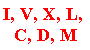CC equals 100 in Roman numerals.calculatorA calculator is a machine that solves math problems. calculusCalculus is a branch of mathematics that was developed separately by Gottfried Wilhelm Leibniz and Isaac Newton in the 1600s. Calculus is used to calculate motion and areas (and many other things). Differentiation and integrations are two major areas of calculus.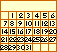calendarA calendar divides the year into periods and lets you know what day it is.

cardinal number

A cardinal number is a whole number that tells you "How many?".
Even Odd 0,2,4 1,3 6,8,10 5,7,9

Carroll diagram

A Carroll diagram (named for the mathematician/author Lewis Carroll) is a rectangular chart that is used to sort items with respect to one, two or three binary (yes/no) categories.carry

When you carry in addition, the sum of the numbers is ten or more, and the ten's place is "carried" to the next place.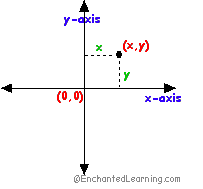Cartesian coordinates

A Cartesian coordinate system is a rectangular coordinate system with two axes (x is the horizontal axis and y is the vertical axis). Every point on the plane can be located by an ordered pair (x,y), which notes its distance from the x-axis and from the y-axis. The axes meet at the origin, the point (0,0). It is named for René Descartes.centCent is another word for penny. center of massThe center of mass is the location at which the entire mass of an object (or set of objects) may be considered for purposes of calculations. It is the point of the average weighted position in space of an object (or a collection of objects). centiCenti is a prefix that means one-hundredth. For example, a centimeter is one hundredth of a meter.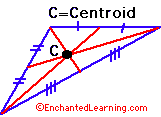centroidThe centroid is the point at which a geometric figure balances. The centroid of a triangle is the point at which the three medians intersect.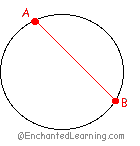chordA chord is a line segment that connects two points on a circle. The longest chord on a circle is the diameter of the circle.circleAll of the points on a circle are the same distance from the center of the circle (that distance is called the radius of the circle).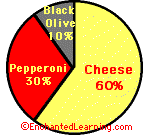circle graphA circle graph (also called a pie chart) is a diagram that is useful for displaying information about the percentages or parts of a whole. circumferenceThe circumference is the distance around an object. The circumference of a circle of radius r is 2πr .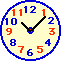clockA clock tells you what time it is. coefficientIn an expression or equation, a coefficient is a number in front of a variable. For example, in the expression 8x, the coefficient is 8 (and the variable is x).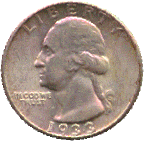coinA coin is a piece of metal money. collinearPoints that are collinear are on the same line.
 a x b = b x acommutative propertyThe commutative property states that for some operations, you can change the order of the terms and not change the outcome of the operation. For example, a x b = b x a and a + b = b + a. The commutative property holds for addition and multiplication; it does not hold for subtraction or division.compassA compass is a device that always points north. A compass is also a device that draws circles.compass roseA compass rose is a design on a map that shows direction. It points which way is north, south, east, west, and some intermediate directions on the map. compatible numbersTwo compatible numbers form a division fact. For example, since 72 divided by 9 is 8, 72 and 9 are compatible numbers (so are 72 and 8).
 complementary angleA complementary angle is an angle that, when added to another angle, equals 90 degrees. complex fractionA complex fraction is a fraction that has a fraction in the numerator, denominator, or both. complex numberA complex number is a number that can be written in the form a + bi, where a and b are real numbers and i is the square root of -1 (an imaginary number ). composite numberA composite number is a number that has more than two factors. For example, 6 is a composite number because it has four factors (1, 2, 3, 6). 5 is not a composite number because it has only 2 factors, 1 and 5.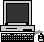computerYou are using a computer right now. concentric circlesConcentric circles are circles that have the same center point. conditionalA conditional (also called an if-then statement or an implication) is a statement of the form, "if p then q" - p is called the antecedent, p is called the consequent. The conditional "if p then q" is false only when p is true and q is false.coneA cone is a shape that has a point at the center and a circular cross-section throughout (it looks like two infinitely-long ice cream cones with the vertices touching). The volume of a single portion of a cone is πr3L/3 (where r=radius of cone at the larger end, L=length of the cone).
 congruentCongruent means having the same shape and size. For example, two triangles are congruent if they have the same interior angles and same side length. Two line segments are congruent if they are the same length. conic sectionA conic section is set of points that results from the intersection of a cone and a plane. Some conic sections include a point, ellipse, circle, parabola, and hyperbola. conjectureA conjecture is an educated guess.connect-the-dotsWhen you do a connect-the-dots puzzle, you draw a line from number to number to make a picture.
 consecutiveConsecutive means in order. For example, 1 and 2 are consecutive whole numbers; 6 and 8 are consecutive even numbers.coordinatesCoordinates are an ordered pair of numbers that show the location of a point on the x-y plane. Every point on the plane can be located by a pair of coordinates (x,y), which notes its distance from the x-axis and the y-axis. cosecantCosecant (abbreviated csc) is a trigonometric ratio, corresponding to the length of the hypotenuse divided by the length of the opposite side of the right triangle. Cosecant is equal to 1/sine. cosineCosine (abbreviated cos) is a trigonometric ratio, corresponding to the length of the adjacent side divided by the length of the hypotenuse of the right triangle.
 cotangentCotangent (abbreviated cot) is a trigonometric ratio, corresponding to the length of the adjacent side divided by the length of the opposite side of the right triangle. Cotangent is equal to 1/tangent. counting numberThe counting numbers are the positive whole numbers, 1, 2, 3, 4, 5... .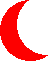crescentA crescent is the shape of the moon shortly before and after the time of the new moon.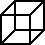cubeA cube is a solid geometric figure with six square faces. The volume of a cube is s3 (where s=length of a side of the cube). The surface area of a cube is 6s2 (where s=length of a side of the cube).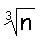cube rootThe cube root of a number, n, is a number whose cube is that number. For example, the cube root of 8 is 2, since 2 x 2 x 2 = 8. In general, the cube root of n is a if a3=n. cubedCubed means raised to the third power, like x3. For example, 2 cubed = 2 x 2 x 2 = 8. cubic equationA cubic equation is an equation whose highest degree term is 3. For example, 5x3 - 8x2 + 2xy = 4 is a cubic equation.curveA curve is a wavy line.cylinderA cylinder is a 3-dimensional figure with a long tube-like body and congruent, parallel bases. A right-circular cylinder has a base that is perpendicular to the axis of the cylinder (it is like a can of food). The volume of a right-circular cylinder is πr2L (where L=length of cylinder, r=radius of cylinder). The surface area of a cylinder is 2πrL + 2πr2 (where L=length of cylinder, r=radius of cylinder).

 +, - EnchantedLearning.comMath Dictionary x, ÷
 A B C D E F G H I J K L M N O P Q R S T U V W X Y Z
 Number Line Fractions Decimals Graphs Measurement Rounding

Click on an underlined word for more information on that subject. If the math term you are looking for is not in the dictionary, please e-mail us.

Enchanted Learning®
Over 35,000 Web Pages
Sample Pages for Prospective Subscribers, or click below

 Overview of Site What's New Enchanted Learning Home Monthly Activity Calendar Books to Print Site Index K-3 Crafts K-3 Themes Little ExplorersPicture dictionary PreK/K Activities Rebus Rhymes Stories Writing Cloze Activities Essay Topics Newspaper Writing Activities Parts of Speech Fiction The Test of Time iPhone app TapQuiz Maps - free iPhone Geography Game Biology Animal Printouts Biology Label Printouts Biomes Birds Butterflies Dinosaurs Food Chain Human Anatomy Mammals Plants Rainforests Sharks Whales Physical Sciences: K-12 Astronomy The Earth Geology Hurricanes Landforms Oceans Tsunami Volcano Languages Dutch French German Italian Japanese (Romaji) Portuguese Spanish Swedish Geography/History Explorers Flags Geography Inventors US History Other Topics Art and Artists Calendars College Finder Crafts Graphic Organizers Label Me! Printouts Math Music Word Wheels

## Enchanted Learning Search

 Search the Enchanted Learning website for: